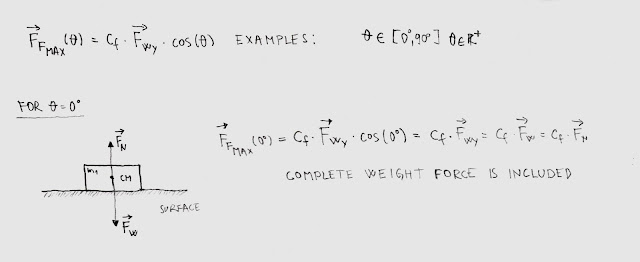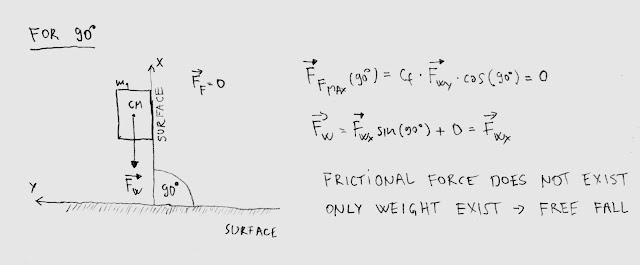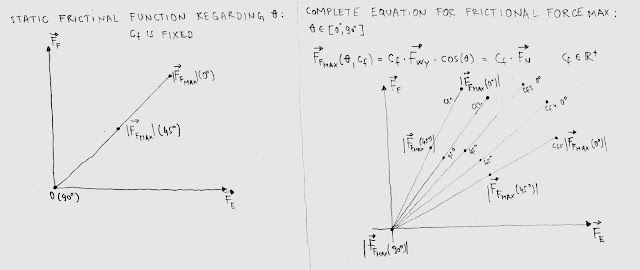### Feel the force around you – Frictional force Part II

This is the second article in a series intended to explain the force of friction. In this article we will see that the maximum value of the static friction force and normal force as well, depends on the incline angle. We will see three different examples in order to explain dependencies between forces and inclines.

Example #1: This is the first example related to cubically shape object with mass m1 standing on the ground surface. In this case the weight force is perpendicular to the ground while reactional normal force acts in the opposite equilibrium direction, where incline angle is zero. Maximum static frictional force in this case, is equal to the maximum value of the normal force (weight) multiplied by the frictional coefficient Cf between two surfaces: ground and cubically shaped object with mass m1.Example #1

Example #2: This is the second example, much more interesting because we will see that mass has dual behavior depending on the incline angle. In this case the inclination angle is set to 45 degrees as it is presented on bellow picture. As we can see, the weight force has composite nature contained of two different forces: cosine part of the weight force and sinus part of the weight force. Cosine part of the weight force is downforce and push the cubically shaped object to the ground, at the same time generating reactional force called the normal force which acts in an opposite equilibrium direction. Pay attention that the normal force is no longer related to complete weight of the object. Instead, it is only related to the cosine part of the weight. What does that mean? That means that the normal force is not a constant, and because of that, it is included in calculations related to the maximum value of the static frictional force. That also means that maximum static friction depends on incline angle as well, and it is not a constant as weight force is. Sinus part of the weight is pulling force with tendencies to pull cubically shaped object to the ground surface. At this point, we will see in the upcoming articles, that we have two possible scenarios: cubically shaped object will slide down or not, which depends on the coefficient of friction between incline and cubically shaped objects. Cosine and sinus part of the weight are embedded forces of the mass where cosine part always acts perpendicular to the surface while sinus part always acting as a pulling force. In this example, we can see that half of the mass is related to a downforce while the other half of the mass is related to the pulling force, which could lead to the object sliding or not.Example #2

Example #3: This is the third and the last example and covers an incline angle set to 90 degrees. In this case the normal force does not exist as well as pulling force, as we can see from bellow equations. There is only a weight force which leads object of the mass m1 to the free fall. At the same time normal force is equal to zero as well as frictional force. When an object touches the ground surface, we will have the same situation as in the example #1.Example #3
Last three examples could lead us to conclude that normal force as well as frictional force are not a constant. Instead, both forces depend on incline angle and we should be aware about that because the movement of the mobile robot could be related to different terrain configurations, a combination of smooth surfaces and inclined surfaces as well (important part for choosing right DC motor). Also, as it is presented, maximum value of the normal force decreases while incline angle becomes higher. The same stand for the maximum static frictional force. As we can see from bellow diagrams, it is a complete set of linear functions against different coefficient of frictions and different incline angles.Linear function against coefficient of friction and different angles

Related articles:
Feel the force around you – Normal force
Normal force - Real catalog examples and calculations
Feel the force around you – Frictional force Part I
Feel the force around you – Frictional force Part III
Feel the force around you – Frictional force Part IV